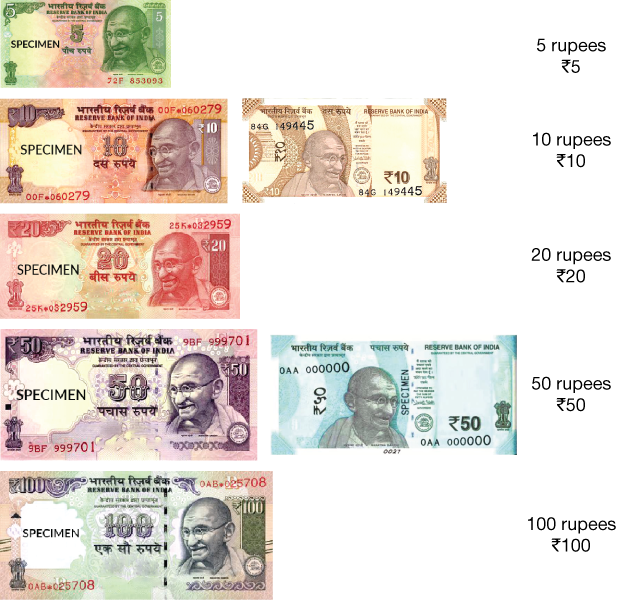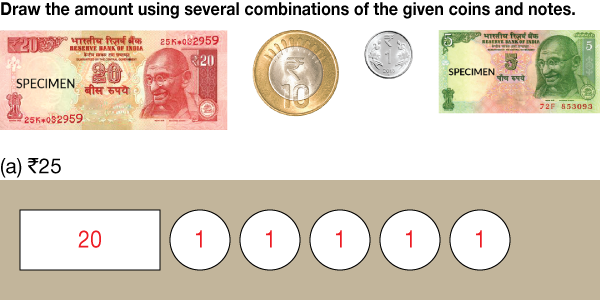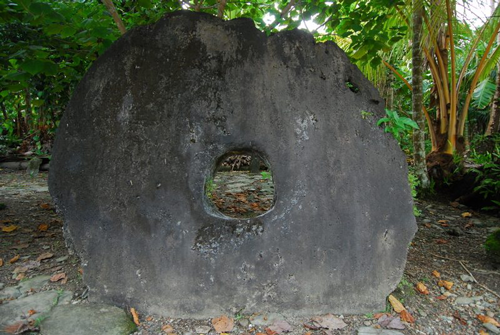# Decomposing Money

Go back to  'Money'

## Introduction to Decomposing Money

When we were trying to understand the denominations of money, we discussed the example of how a shopkeeper would repay you the change after you bought something from him. That is just one example of how we can break down a sum of money into its smaller denominations. Just like we decompose a number into its components of different place values, similarly money can also be decomposed into smaller units.## The Big Idea: Decomposing Money

### A simple idea: What is decomposition of money?

Let us take the example of a Rs. 2000 currency note. It represents a value of Rs. 2000, and it has a declaration from the central bank that it promises to pay that amount to the bearer of that currency note. We know that below Rs. 2000, the other denominations are 500, 200, 100, 50, 20, 10, 5 and 1. If we just consider the bigger denominations of 500, 200 and 100, then these are some ways in which Rs. 2000 can be broken into:

\begin{align} & {\text{2000 = 4}} \times {\text{500}}\\ & {\text{2000 = 10}} \times {\text{200}} \\ & {\text{2000 = 20}} \times {\text{100}}\\ \end{align}

If we use combinations of 500, 200 and 100, then Rs. 2000 can be broken into

\begin{align} &2000 =\\ &\left( {3 \times 500} \right) + \left( {2 \times 200} \right) + \left( {1 \times 100} \right)\\ & 2000 =\\& \left( {2 \times 500} \right) + \left( {4 \times 200} \right) + \left( {2 \times 100} \right)\\ & {\rm{ \ldots }}{\text{.and so on.}} \end{align}

## How is it important?

### The purpose of decomposition of money

Understanding the decomposition of money would help you to get a grip on the basic mathematics involved in the handling of money. This would equip you with the tools to handle money better. Money is something that sees the principles of mathematics being applied in daily life.When you wish to find out a fraction, you need to know how to decompose money. So, if your grandfather has Rs.1000 and he wants to give equal parts of it to each of his five grandchildren, then how much would you and your four cousins get? You would get \begin{align}\frac{1}{5} \times 1000 = {\text{Rs}}.200\end{align}. If there were 4 grandchildren instead of 5, then each child would get Rs.250.

How about when you wish to understand how to get back change when you buy something. When you buy something for Rs.165, then these are the amounts you would get back if you pay different denominations:

\begin{align} &\text{When you give Rs.200 note:}\\& \text{You can get back Rs.35}\\ &= 20 + 10 + 5\\\\ &\text{When you give Rs.500 note:} \\& \text {You can get back Rs.335}\\& = 200 + 100 + 10 + 10 + 10 + 5\\\\ &\text{When you give Rs.2000 note:} \\ &\text{You can get back Rs.1835} \\&= 500 + 500 + 500 + 200 + 100 + 20 + 10 + 5 \end{align}

### The future of monetary transactions

We talk about understanding the denomination of money and also decomposing it. However, in the present day, we often carry out transactions using money without actually seeing or touching it. Transactions between countries are carried out on the basis of gold, but the gold is not actually physically transferred. As individuals, your parents would do most of their financial transactions (like paying the rent or paying for groceries) online, without actually counting out the money. By the time you are ready to earn and spend your own money, almost every transaction would be online.

### An Interesting Kind of Money

There is an island called Yap which is part of the present-day Federated States of Micronesia. Till the early 1800s, it hadn’t been touched by civilization. But they had a well-developed financial system using a round stone (of diameter 12 feet) called rai as the unit of currency. The round stone had a hole in the centre through which a pole could be inserted in order to transport it. Transactions were measured as fractions of a rai, and bigger values would be represented by 1 rai. All outstanding debts would be carried forward in expectation of some future exchange!